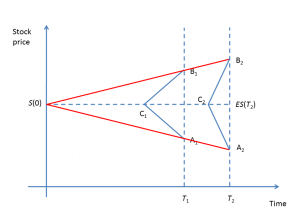23
Feb 18

## Call options and probabilistic intuition - dependence on time## Call options and probabilistic intuition - dependence on time

As before, we change one variable - in this case it's time to expiration$T$, while holding others fixed, and see how the call price changes. We've assumed that at each$T$, the stock price distribution is triangular, symmetric and centered at$S(0)$. This time on top of that assumption we add the following.

Assumption 2. The standard deviation of the stock price distribution is proportional to$T$.

This standard deviation is a measure of riskiness and unpredictability of the stock price. The farther into the future, the more difficult it is to predict.

## Areas of influence of the initial stock priceFigure 1. Areas of influence for two times

As a result of our assumptions, we can visualize areas of influence for two times in Figure 1. Points$S(0),\ A_1,\ A_2$ are on the same straight line. Similarly, points$S(0),\ B_1,\ B_2$ are on the same straight line. This is because by Assumption 2, the triangles$\triangle S(0),A_1,B_1$ and$\triangle S(0),A_2,B_2$ are similar. Note that the triangle$\triangle A_2, B_2,C_2$ is flatter than$\triangle A_1, B_1,C_1$ because in both cases the area should be 1.

## Finding ranges of initial stock prices that can lead to ITM areasFigure 2. Initial price ranges

In Figure 2 we find initial price ranges that may contribute to the ITM areas. For example, for time$T_1$ we find the highest initial price$S_1$ such that its area of influence doesn't intersect the ITM area$\{K. Only initial prices above$S_1$ can contribute to$\{K (such prices are shown by the green arrow). Similarly, only initial prices above$S_2$ can contribute to$\{K (such prices are shown by the blue arrow). Since the blue area is wider than the green area, the call expiring at$T_2$ must be more expensive than the one expiring at$T_1$.

## Fast time decay of OTM optionFigure 3. Call price as a function of time to expiration

If an OTM option continues to be OTM as expiration approaches, the likelihood of it becoming ITM quickly declines and the call price erodes to zero. This is illustrated in Figure 3 (from Mathematica), where time is measured in years, so the chart traces the call price as time to expiration declines from half a year to zero. The practical recommendation is to sell the option before it dies on you, if the odds of the stock moving higher are low.